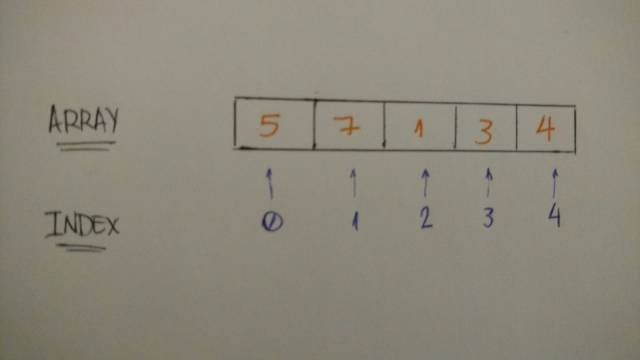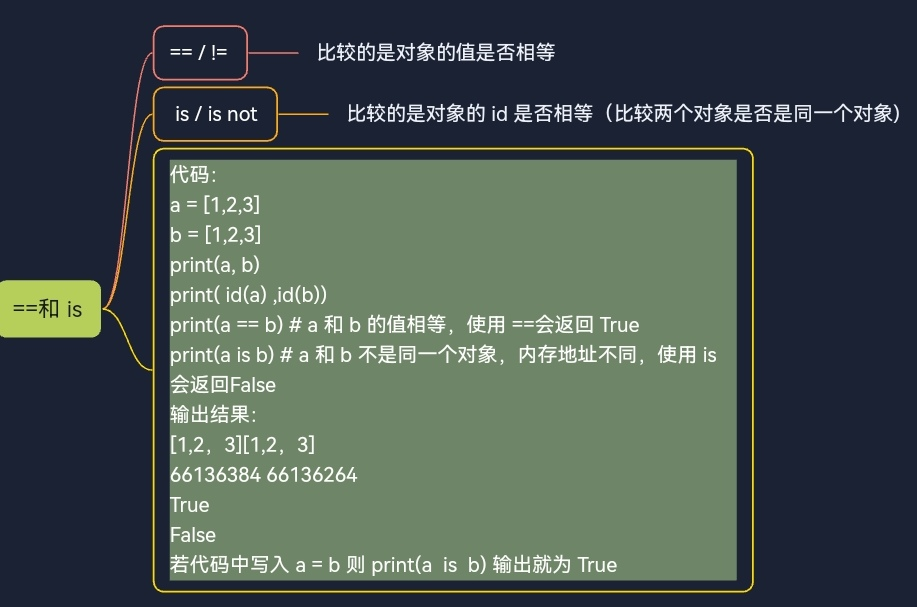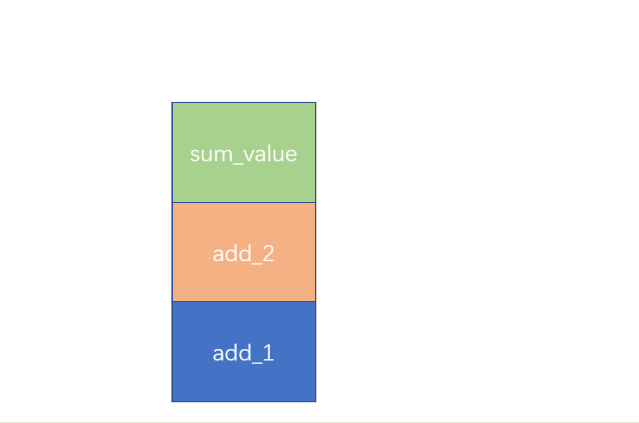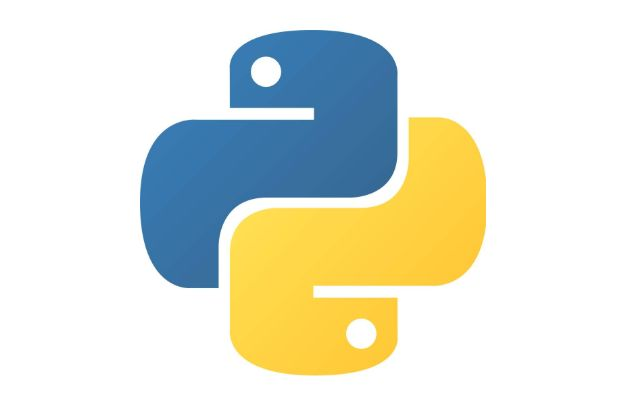# 从 Zero 到 Hero ，一文掌握 Python

+关注继续查看

Python 基础

1.变量

Python 中定义一个变量并为它赋值是很容易的。假如你想存储数字 1 到变量 “one” ，让我们试试看：

one = 1

two = 2

some_number = 10000

# booleanstrue_boolean = Truefalse_boolean = False# stringmy_name = "Leandro Tk"# floatbook_price = 15.80

2.控制流程：条件语句

“If” 使用一个表达式来判断一个语句是 True 还是 False ，如果是 True ，那么执行 if 内的代码，例子如下：

if True:

print("Hello Python If")if 2 > 1:

print("2 is greater than 1")

2 比 1 大，所以 print 代码被执行。

if 1 > 2:

print("1 is greater than 2")else:

print("1 is not greater than 2")

1 比 2 小，所以 “else” 里面的代码会执行。

if 1 > 2:

print("1 is greater than 2")elif 2 > 1:

print("1 is not greater than 2")else:

print("1 is equal to 2")

3. 循环和迭代

While 循环：当语句是 True 时，while 内部的代码块会执行。所以下面这段代码会打印出 1 到 10 。

num = 1

while num <= 10:

print(num)

num += 1

while 循环需要循环条件，如果条件一直是 True ，它将会一直迭代，当 num 的值为 11 时，循环条件为 false 。

loop_condition = Truewhile loop_condition:

print("Loop Condition keeps: %s" %(loop_condition))

loop_condition = False

For 循环：你可以在代码块上应用变量 “num” ，而 “for” 语句将为你迭代它。此代码将打印与 while 中相同的代码：从 1 到 10 。

for i in range(1, 11):

print(i)

List：集合 | 数组 | 数据结构

my_integers = [1, 2, 3, 4, 5]my_integers = [5, 7, 1, 3, 4]

print(my_integers) # 5print(my_integers) # 7print(my_integers) # 4

relatives_names = [  "Toshiaki",  "Juliana",  "Yuji",  "Bruno",  "Kaio"]

print(relatives_names) # Kaio

bookshelf = []

bookshelf.append("The Effective Engineer")

bookshelf.append("The 4 Hour Work Week")

print(bookshelf) # The Effective Engineerprint(bookshelf) # The 4 Hour Work W

dictionary_example = {

"key1""value1",

"key2""value2",

"key3""value3"

}

Key 是指向 value 的索引。我们如何访问字典中的 value 呢？你应该猜到了，那就是使用 key 。我们试一下：

dictionary_tk = {

"name""Leandro",

"nickname""Tk",

"nationality""Brazilian"

}

print("My name is %s" %(dictionary_tk["name"])) # My name is Leandro

print("But you can call me %s" %(dictionary_tk["nickname"])) # But you can call me Tk

print("And by the way I'm %s" %(dictionary_tk["nationality"])) # And by the way I'm Brazilian

dictionary_tk = {

"name""Leandro",

"nickname""Tk",

"nationality""Brazilian",

"age"24

}

print("My name is %s" %(dictionary_tk["name"])) # My name is Leandro

print("But you can call me %s" %(dictionary_tk["nickname"])) # But you can call me Tk

print("And by the way I'm %i and %s" %(dictionary_tk["age"], dictionary_tk["nationality"])) # And by the way I'm Brazilian

bookshelf = [

"The Effective Engineer",

"The 4 hours work week",

"Zero to One",

"Lean Startup",

"Hooked"

]

for book in bookshelf:

print(book)

dictionary = { "some_key""some_value" }

for key in dictionary:

print("%s --> %s" %(key, dictionary[key])) # some_key --> some_value

dictionary = { "some_key""some_value" }

for key, value in dictionary.items():

print("%s --> %s" %(key, value))# some_key --> some_value

dictionary_tk = {

"name""Leandro",

"nickname""Tk",

"nationality""Brazilian",

"age"24

}

for attribute, value in dictionary_tk.items():

print("My %s is %s" %(attribute, value))

# My name is Leandro

# My nickname is Tk

# My nationality is Brazilian

# My age is 24

Python 面向对象编程模式：ON

Python，作为一种面向对象编程语言，存在这样的概念：类和对象。

class Vehicle:

pass

car = Vehicle()

print(car) # <__main__.Vehicle instance at 0x7fb1de6c2638>

class Vehicle:

def __init__(self, number_of_wheels, type_of_tank, seating_capacity, maximum_velocity):

self.number_of_wheels = number_of_wheels

self.type_of_tank = type_of_tank

self.seating_capacity = seating_capacity

self.maximum_velocity = maximum_velocity

tesla_model_s = Vehicle(4, 'electric', 5, 250)

class Vehicle:

def __init__(self, number_of_wheels, type_of_tank, seating_capacity, maximum_velocity):

self.number_of_wheels = number_of_wheels

self.type_of_tank = type_of_tank

self.seating_capacity = seating_capacity

self.maximum_velocity = maximum_velocity    def number_of_wheels(self):

return self.number_of_wheels    def set_number_of_wheels(self, number):

self.number_of_wheels = number

class Vehicle:

def __init__(self, number_of_wheels, type_of_tank, seating_capacity, maximum_velocity):

self.number_of_wheels = number_of_wheels

self.type_of_tank = type_of_tank

self.seating_capacity = seating_capacity

self.maximum_velocity = maximum_velocity    @property

def number_of_wheels(self):

return self.number_of_wheels    @number_of_wheels.setter

def number_of_wheels(self, number):

self.number_of_wheels = number

tesla_model_s = Vehicle(4, 'electric', 5, 250)

print(tesla_model_s.number_of_wheels) # 4tesla_model_s.number_of_wheels = 2 # setting number of wheels to 2print(tesla_model_s.number_of_wheels) # 2

class Vehicle:

def __init__(self, number_of_wheels, type_of_tank, seating_capacity, maximum_velocity):

self.number_of_wheels = number_of_wheels

self.type_of_tank = type_of_tank

self.seating_capacity = seating_capacity

self.maximum_velocity = maximum_velocity    def make_noise(self):

print('VRUUUUUUUM')

tesla_model_s = Vehicle(4, 'electric', 5, 250)

tesla_model_s.make_noise() # VRUUUUUUUM

“封装可被用于隐藏数据成员和成员函数。按照这个定义，封装意味着 对象的内部表示一般在对象定义的外部视图中隐藏。” — Wikipedia

class Person:

def __init__(self, first_name):

self.first_name = first_name

tk = Person('TK')

print(tk.first_name) # => TK

class Person:

first_name = 'TK'

tk = Person()

print(tk.first_name) # => TK

tk = Person('TK')

tk.first_name = 'Kaio'print(tk.first_name) # => Kaio

Non-public实例变量

“除了从对象内部外无法被访问的‘Private’实例变量在Python中并不存在。然而，这里有一个多数Python代码都会遵守的惯例：使用下划线作为前缀的命名(例如 _spam)应该被认为是API的非公开部分（不管是函数、方法还是数据成员）” —  Python软件基础

class Person:

def __init__(self, first_name, email):

self.first_name = first_name

self._email = email

tk = Person('TK', 'tk@mail.com')

print(tk._email) # tk@mail.com

class Person:

def __init__(self, first_name, email):

self.first_name = first_name

self._email = email    def update_email(self, new_email):

self._email = new_email    def email(self):

return self._email

tk = Person('TK', 'tk@mail.com')

print(tk.email()) # => tk@mail.comtk._email = 'new_tk@mail.com'print(tk.email()) # => tk@mail.comtk.update_email('new_tk@mail.com')

print(tk.email()) # => new_tk@mail.com

1. 我们使用first_name TK和email tk@mail.com初始化了一个新对象

2. 使用方法访问非公开变量email并输出它

3. 尝试在类外部设置一个新的email

4. 我们需要将非公开变量视为API的非公开部分

5. 使用我们的实例方法来更新非公开变量

6. 成功!我们使用辅助方法在类内部更新了它。

class Person:

def __init__(self, first_name, age):

self.first_name = first_name

self._age = age    def show_age(self):

return self._age

tk = Person('TK', 25)

print(tk.show_age()) # => 25

class Person:

def __init__(self, first_name, age):

self.first_name = first_name

self._age = age    def _show_age(self):

return self._age

tk = Person('TK', 25)

print(tk._show_age()) # => 25

class Person:

def __init__(self, first_name, age):

self.first_name = first_name

self._age = age    def show_age(self):

return self._get_age()    def _get_age(self):

return self._age

tk = Person('TK', 25)

print(tk.show_age()) # => 25

class Car:

def __init__(self, number_of_wheels, seating_capacity, maximum_velocity):

self.number_of_wheels = number_of_wheels

self.seating_capacity = seating_capacity

self.maximum_velocity = maximum_velocity

my_car = Car(4, 5, 250)

print(my_car.number_of_wheels)

print(my_car.seating_capacity)

print(my_car.maximum_velocity)

class ElectricCar(Car):

def __init__(self, number_of_wheels, seating_capacity, maximum_velocity):

Car.__init__(self, number_of_wheels, seating_capacity, maximum_velocity)

my_electric_car = ElectricCar(4, 5, 250)

print(my_electric_car.number_of_wheels) # => 4

print(my_electric_car.seating_capacity) # => 5

print(my_electric_car.maximum_velocity) # => 250

• Python 变量是如何工作的

• Python 条件语句是如何工作的

• Python 循环（ while 和 for ）是如何工作的

• 如何使用链表：Collection |Array

• 字典式键值集合

• 我们如何遍历这些数据结构

• 对象和类

• 作为对象数据的属性

• 作为对象行为的方法

• 使用 Python getter/setter 以及属性修饰器

• 封装：隐藏信息

• 继承：行为和特征Python中的==和 is
Python中的==和 is自制脑图 == / !=比较的是对象的值是否相等。 is / is not比较的是对象的 id 是否相等（比较两个对象是否是同一个对象)。
19 0Python | Python 的 is 和 == 你了解么？

42 079 0784 0[python skill]Python 中 NaN 和 None 的详细比较

1787 0# 01基础篇 # 变量 1 #int 2 one=1 3 some_number=100 4 5 print("one=",one) #print type1 6 print("some_number=",some_number) #python3 print need a...
980 0python class 初学
python class 初学 被测试的 code 我在lib目录创建一个hehe文件夹，和一个__init__空文件。把xclass（我的代码）放进去。xclass： print ('这里在执行') class Student(): def __init__(self,name,age): self.
936 0[Python]Python Class 中的 函数定义中的 self
1280 0Python tips
1354 0

Python 脚本入门21448

Python 脚本进阶13764

【从绘图开始】Python零基础入门86130

Python系列直播第一讲——Python中的一切皆对象

From Python Scikit-Learn to Sc